# Kerala Board Question Paper for SSLC Class 10th Chemistry 2018 In PDF

## KBPE 10th Standard Chemistry Exam Question Paper 2018 with Solutions – Free Download

Kerala Board SSLC Class 10 Chemistry 2018 Question Paper with solutions help students to prepare most effectively for the board exams. This resource is used by students to ace the final exams. The Class 10 exam question paper lays down the foundation for most of the questions that are likely to be asked in the Chemistry paper for the final exam. Students can also download the PDFs of the solved or unsolved question paper, as per their preference by clicking on the links given. For the convenience of the students, we have listed the clickable links in this article. Additionally, we provide the questions and answers on the web-page, as well.

All students who have mastered the entire complex theorems and formulas from Chemistry will find it easier to ace the final exams. These students will also find it easy to understand the question types often asked in the KBPE SSLC Class 10 Chemistry exam. They can solve the previous papers of Kerala Board Class 10 Chemistry. After solving the papers, students can refer to the solutions in order to self-analyse their performance and thus, scale their preparation level for the Class 10 board exams. Doing so will help the students to determine where they have gone wrong while answering the question paper and how they can further avoid these types of errors. Solving these papers helps them to get an overview of the question paper design and the marks’ distribution. Students can also identify the difficulty level of the exams with the help of these SSLC previous year question paper solutions.

### Kerala Board Class 10 Chemistry 2018 Question Paper with Solutions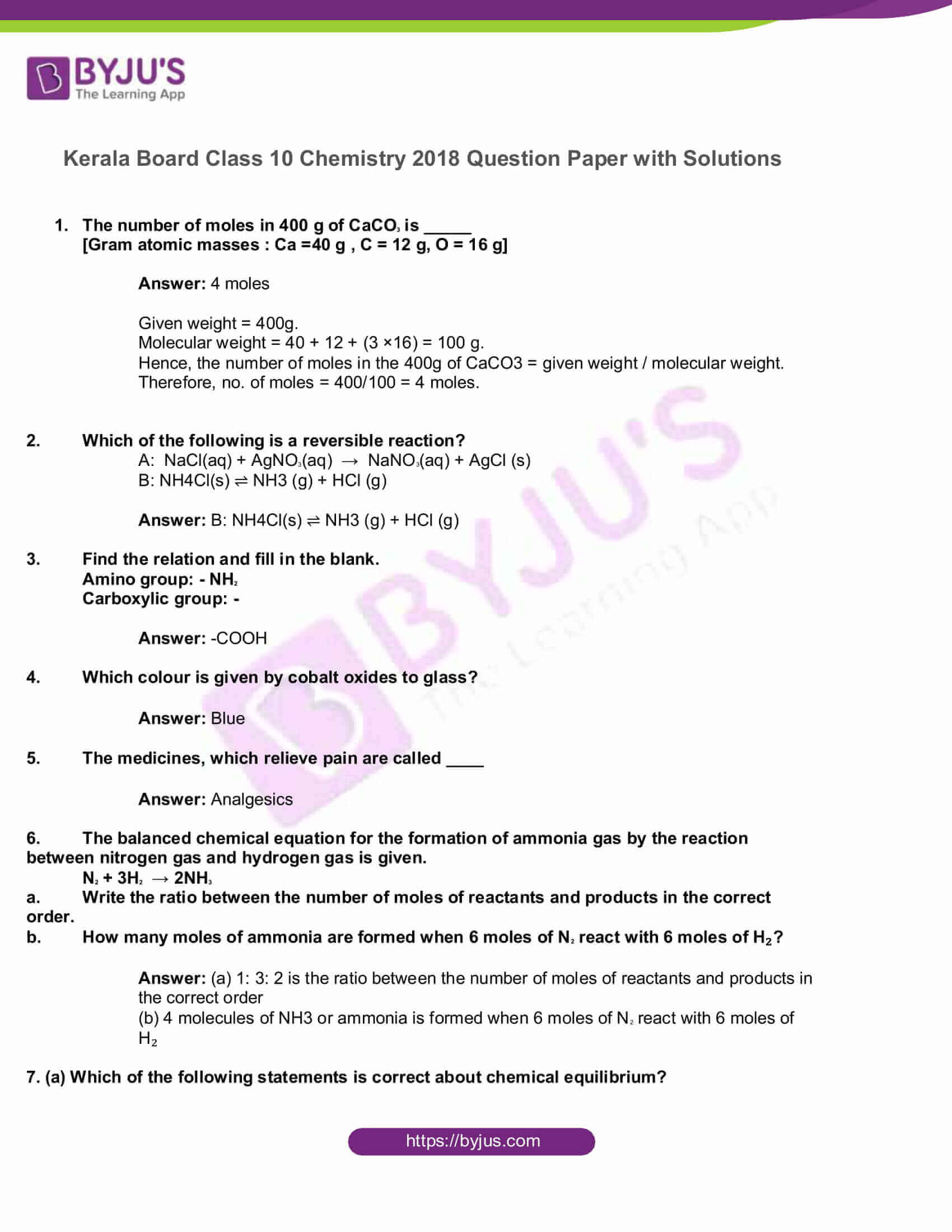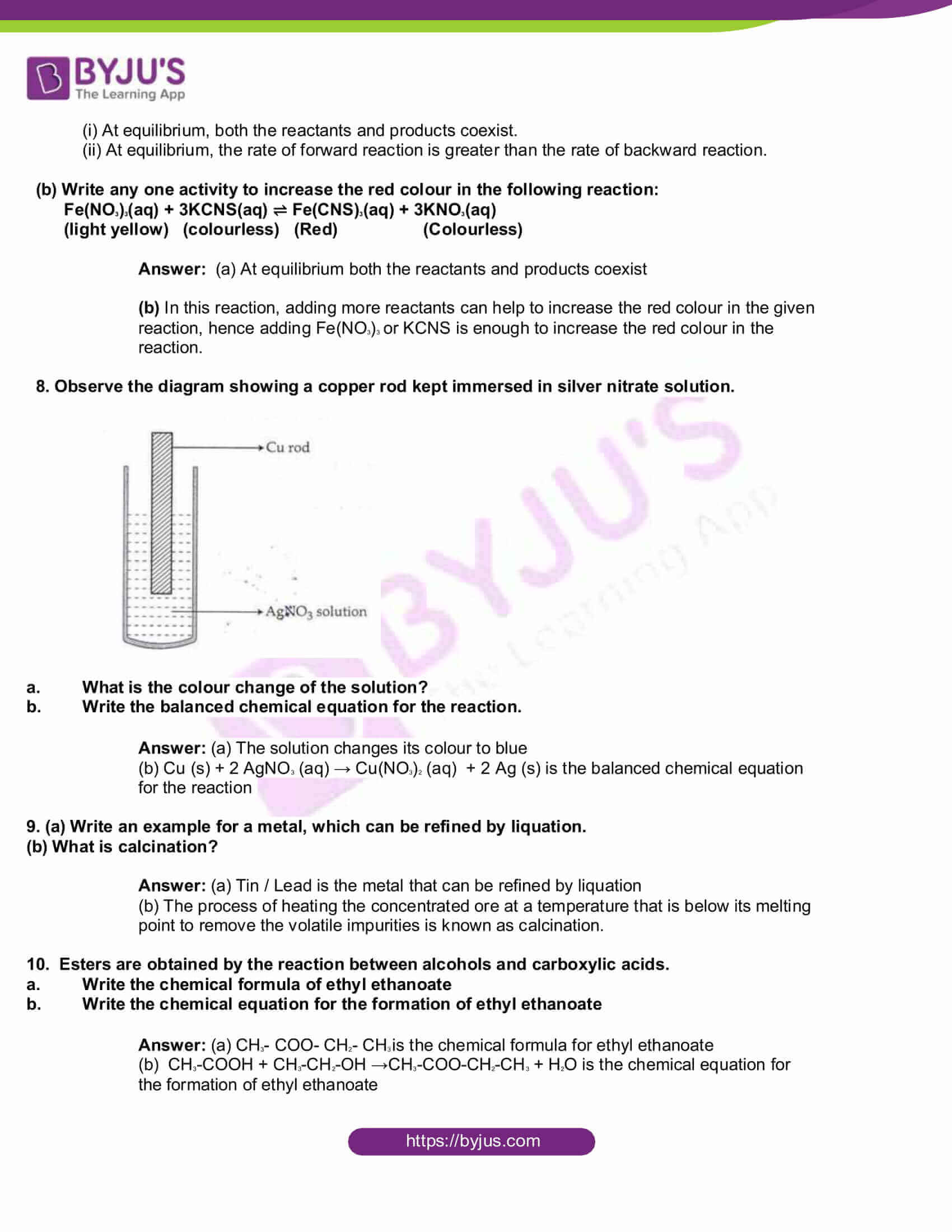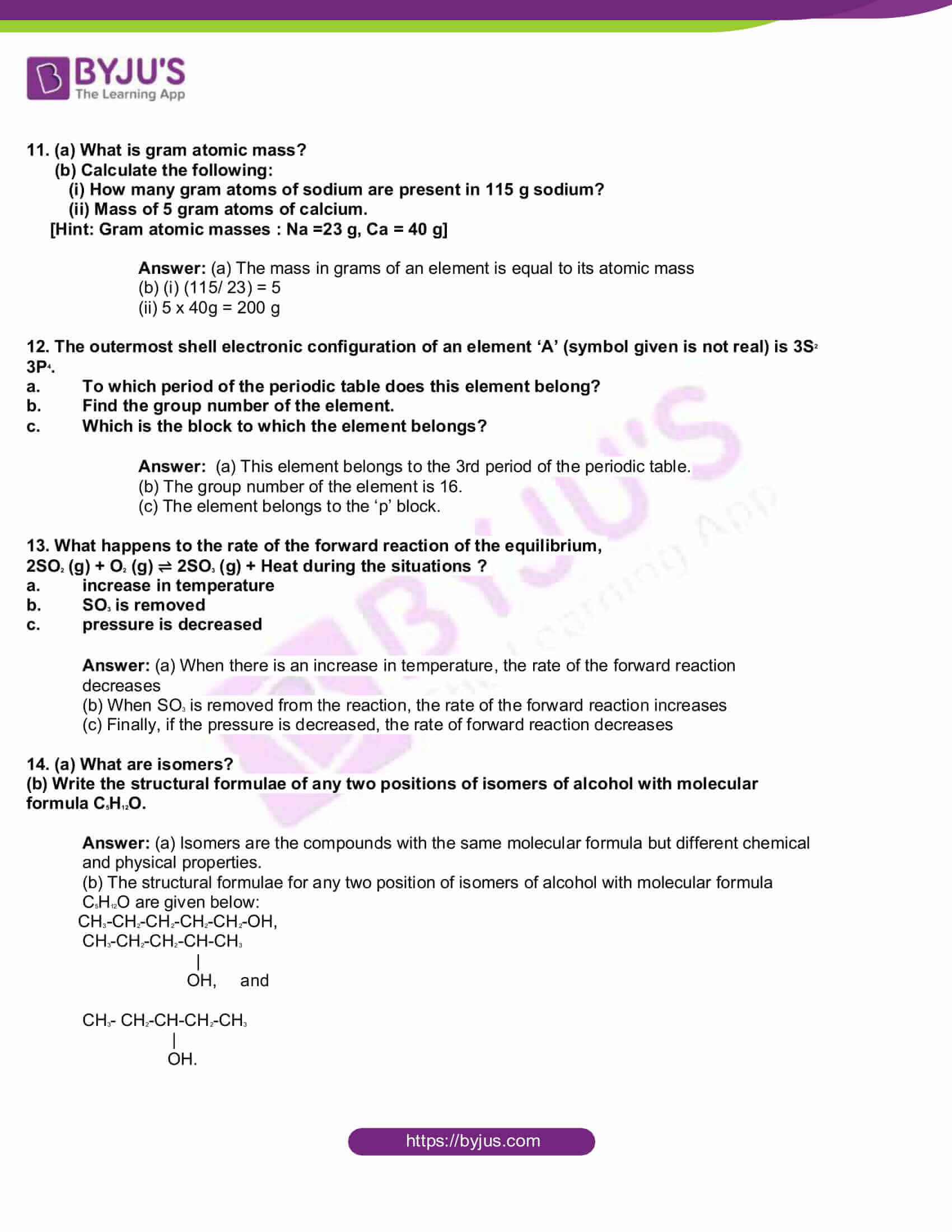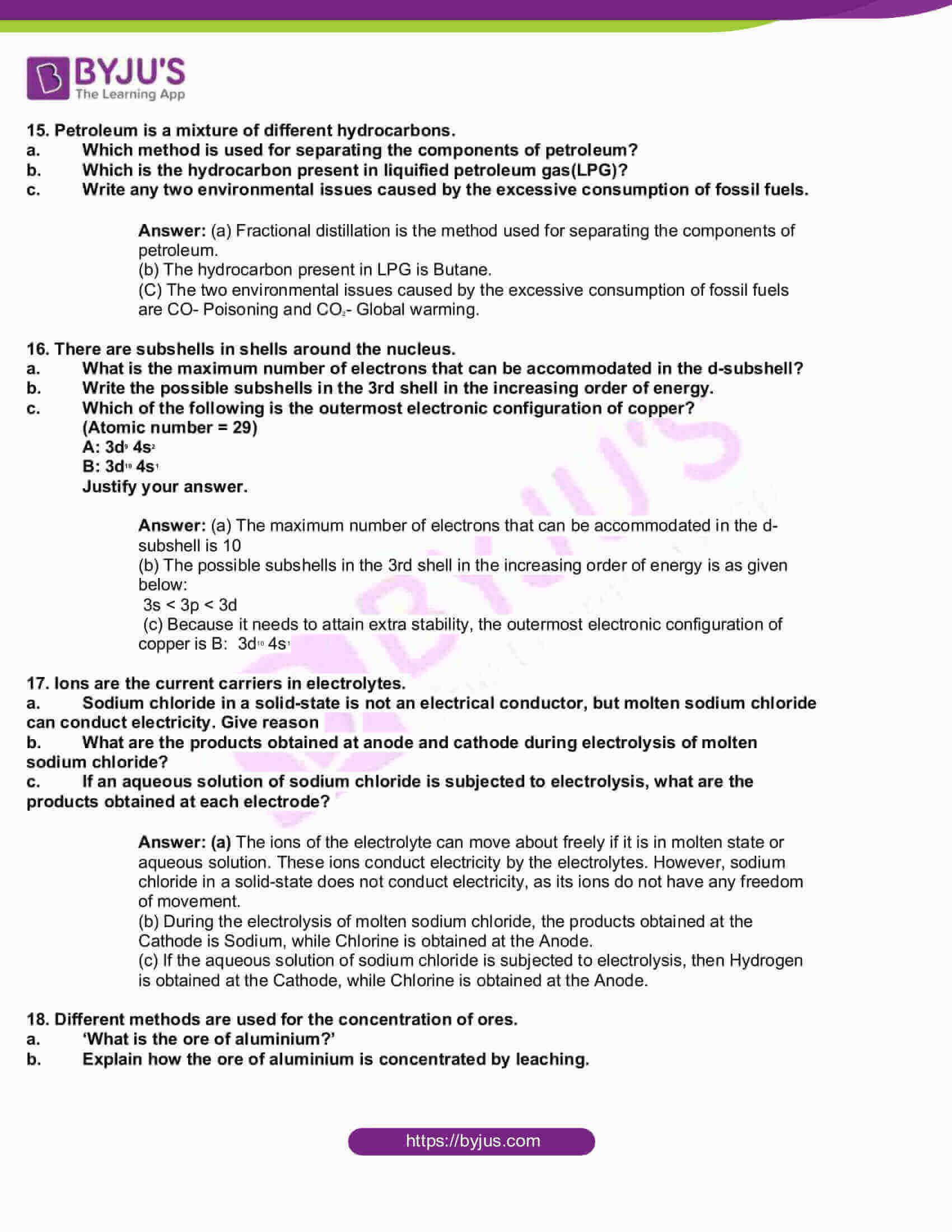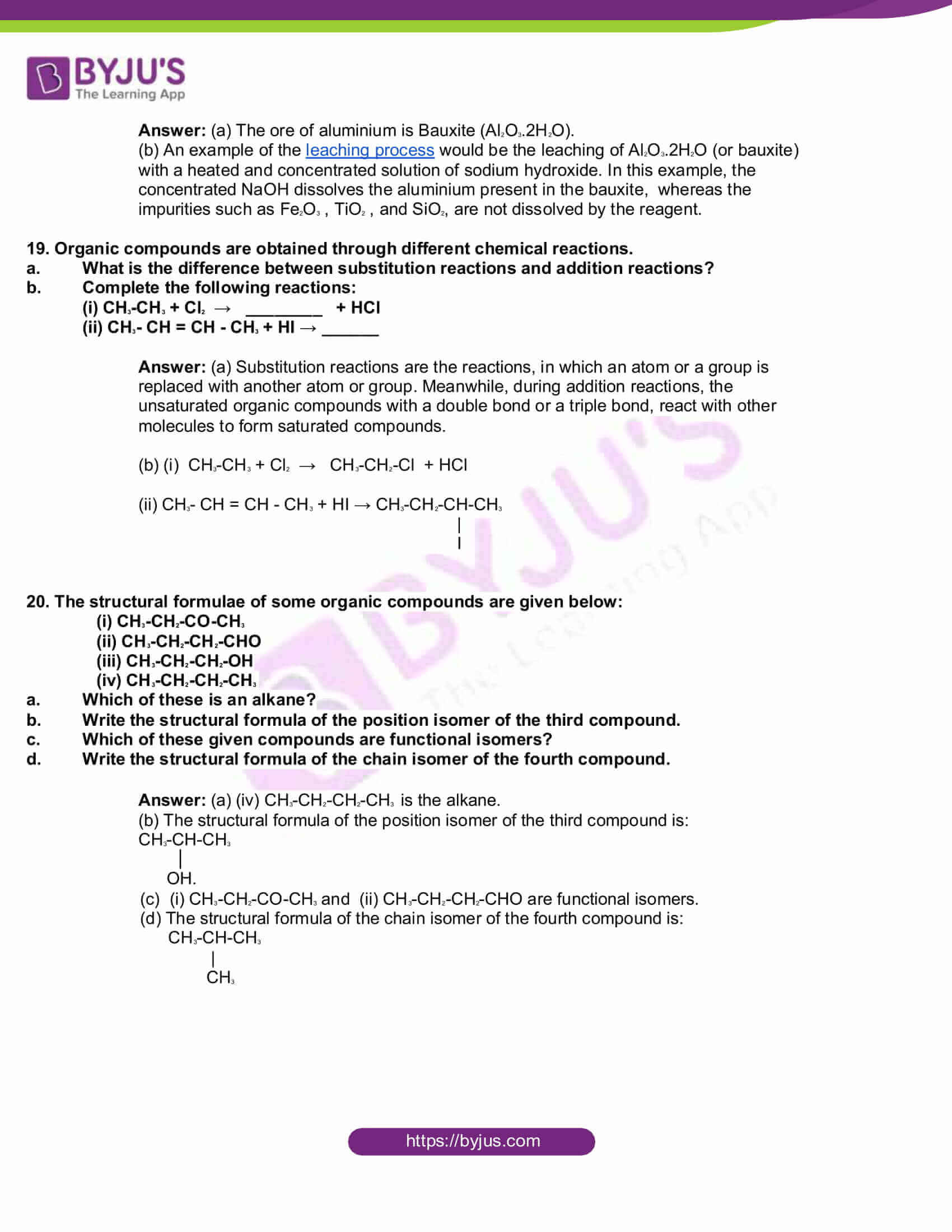1. The number of moles in 400 g of CaCO3 is _____

[Gram atomic masses : Ca =40 g , C = 12 g, O = 16 g]

Given weight = 400g.

Molecular weight = 40 + 12 + (3 ×16) = 100 g.

Hence, the number of moles in the 400g of CaCO3 = given weight / molecular weight.

Therefore, no. of moles = 400/100 = 4 moles.

1. Which of the following is a reversible reaction?

A: NaCl(aq) + AgNO3(aq) → NaNO3(aq) + AgCl (s)

B: NH4Cl(s) ⇌ NH3 (g) + HCl (g)

Answer: B: NH4Cl(s) ⇌ NH3 (g) + HCl (g)

1. Find the relation and fill in the blank.

Amino group: – NH2

Carboxylic group: –

1. Which colour is given by cobalt oxides to glass?

1. The medicines, which relieve pain are called ____

1. The balanced chemical equation for the formation of ammonia gas by the reaction between nitrogen gas and hydrogen gas is given.

N2 + 3H2 → 2NH3

1. Write the ratio between the number of moles of reactants and products in the correct order.
2. How many moles of ammonia are formed when 6 moles of N2 react with 6 moles of H₂?

Answer: (a) 1: 3: 2 is the ratio between the number of moles of reactants and products in the correct order

(b) 4 molecules of NH3 or ammonia is formed when 6 moles of N2 react with 6 moles of H₂

7. (a) Which of the following statements is correct about chemical equilibrium?

(i) At equilibrium, both the reactants and products coexist.

(ii) At equilibrium, the rate of forward reaction is greater than the rate of backward reaction.

(b) Write any one activity to increase the red colour in the following reaction:

Fe(NO3)3(aq) + 3KCNS(aq) ⇌ Fe(CNS)3(aq) + 3KNO3(aq)

(light yellow) (colourless) (Red) (Colourless)

Answer: (a) At equilibrium both the reactants and products coexist

(b) In this reaction, adding more reactants can help to increase the red colour in the given reaction, hence adding Fe(NO3)3 or KCNS is enough to increase the red colour in the reaction.

8. Observe the diagram showing a copper rod kept immersed in silver nitrate solution.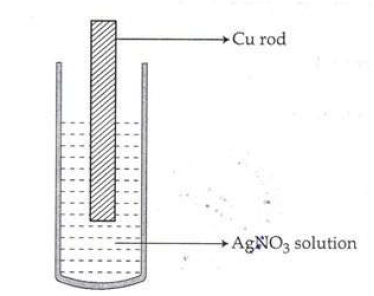1. What is the colour change of the solution?
2. Write the balanced chemical equation for the reaction.

Answer: (a) The solution changes its colour to blue

(b) Cu (s) + 2 AgNO3 (aq) → Cu(NO3)2 (aq) + 2 Ag (s) is the balanced chemical equation for the reaction

9. (a) Write an example for a metal, which can be refined by liquation.

(b) What is calcination?

Answer: (a) Tin / Lead is the metal that can be refined by liquation

(b) The process of heating the concentrated ore at a temperature that is below its melting point to remove the volatile impurities is known as calcination.

10. Esters are obtained by the reaction between alcohols and carboxylic acids.

1. Write the chemical formula of ethyl ethanoate
2. Write the chemical equation for the formation of ethyl ethanoate

Answer: (a) CH3– COO- CH2– CH3 is the chemical formula for ethyl ethanoate

(b) CH3-COOH + CH3-CH2-OH →CH3-COO-CH2-CH3 + H2O is the chemical equation for the formation of ethyl ethanoate

11. (a) What is gram atomic mass?

(b) Calculate the following:

(i) How many gram atoms of sodium are present in 115 g sodium?

(ii) Mass of 5 gram atoms of calcium.

[Hint: Gram atomic masses : Na =23 g, Ca = 40 g]

Answer: (a) The mass in grams of an element is equal to its atomic mass

(b) (i) (115/ 23) = 5

(ii) 5 x 40g = 200 g

12. The outermost shell electronic configuration of an element ‘A’ (symbol given is not real) is 3S2 3P4.

1. To which period of the periodic table does this element belong?
2. Find the group number of the element.
3. Which is the block to which the element belongs?

Answer: (a) This element belongs to the 3rd period of the periodic table.

(b) The group number of the element is 16.

(c) The element belongs to the ‘p’ block.

13. What happens to the rate of the forward reaction of the equilibrium,

2SO2 (g) + O2 (g) ⇌ 2SO3 (g) + Heat during the situations ?

1. increase in temperature
2. SO3 is removed
3. pressure is decreased

Answer: (a) When there is an increase in temperature, the rate of the forward reaction decreases

(b) When SO3 is removed from the reaction, the rate of the forward reaction increases

(c) Finally, if the pressure is decreased, the rate of forward reaction decreases

14. (a) What are isomers?

(b) Write the structural formulae of any two positions of isomers of alcohol with molecular formula C5H12O.

Answer: (a) Isomers are the compounds with the same molecular formula but different chemical and physical properties.

(b) The structural formulae for any two position of isomers of alcohol with molecular formula C5H12O are given below:

CH3-CH2-CH2-CH2-CH2-OH,

CH3-CH2-CH2-CH-CH3

|

OH, and

CH3– CH2-CH-CH2-CH3

|

OH.

15. Petroleum is a mixture of different hydrocarbons.

1. Which method is used for separating the components of petroleum?
2. Which is the hydrocarbon present in liquified petroleum gas(LPG)?
3. Write any two environmental issues caused by the excessive consumption of fossil fuels.

Answer: (a) Fractional distillation is the method used for separating the components of petroleum.

(b) The hydrocarbon present in LPG is Butane.

(C) The two environmental issues caused by the excessive consumption of fossil fuels are CO- Poisoning and CO2– Global warming.

16. There are subshells in shells around the nucleus.

1. What is the maximum number of electrons that can be accommodated in the d-subshell?
2. Write the possible subshells in the 3rd shell in the increasing order of energy.
3. Which of the following is the outermost electronic configuration of copper?

(Atomic number = 29)

A: 3d9 4s2

B: 3d10 4s1

Answer: (a) The maximum number of electrons that can be accommodated in the d-subshell is 10

(b) The possible subshells in the 3rd shell in the increasing order of energy is as given below:

3s < 3p < 3d

(c) Because it needs to attain extra stability, the outermost electronic configuration of copper is B: 3d10 4s1

17. Ions are the current carriers in electrolytes.

1. Sodium chloride in a solid-state is not an electrical conductor, but molten sodium chloride can conduct electricity. Give reason
2. What are the products obtained at anode and cathode during electrolysis of molten sodium chloride?
3. If an aqueous solution of sodium chloride is subjected to electrolysis, what are the products obtained at each electrode?

Answer: (a) The ions of the electrolyte can move about freely if it is in molten state or aqueous solution. These ions conduct electricity by the electrolytes. However, sodium chloride in a solid-state does not conduct electricity, as its ions do not have any freedom of movement.

(b) During the electrolysis of molten sodium chloride, the products obtained at the Cathode is Sodium, while Chlorine is obtained at the Anode.

(c) If the aqueous solution of sodium chloride is subjected to electrolysis, then Hydrogen is obtained at the Cathode, while Chlorine is obtained at the Anode.

18. Different methods are used for the concentration of ores.

1. ‘What is the ore of aluminium?’
2. Explain how the ore of aluminium is concentrated by leaching.

Answer: (a) The ore of aluminium is Bauxite (Al2O3.2H2O).

(b) An example of the leaching process would be the leaching of Al2O3.2H2O (or bauxite) with a heated and concentrated solution of sodium hydroxide. In this example, the concentrated NaOH dissolves the aluminium present in the bauxite, whereas the impurities such as Fe2O3 , TiO2 , and SiO2, are not dissolved by the reagent.

19. Organic compounds are obtained through different chemical reactions.

1. What is the difference between substitution reactions and addition reactions?
2. Complete the following reactions:

(i) CH3-CH3 + Cl2 → ________ + HCl

(ii) CH3– CH = CH – CH3 + HI → ______

Answer: (a) Substitution reactions are the reactions, in which an atom or a group is replaced with another atom or group. Meanwhile, during addition reactions, the unsaturated organic compounds with a double bond or a triple bond, react with other molecules to form saturated compounds.

(b) (i) CH3-CH3 + Cl2 → CH3-CH2-Cl + HCl

(ii) CH3– CH = CH – CH3 + HI → CH3-CH2-CH-CH3

|

I

20. The structural formulae of some organic compounds are given below:

(i) CH3-CH2-CO-CH3

(ii) CH3-CH2-CH2-CHO

(iii) CH3-CH2-CH2-OH

(iv) CH3-CH2-CH2-CH3

1. Which of these is an alkane?
2. Write the structural formula of the position isomer of the third compound.
3. Which of these given compounds are functional isomers?
4. Write the structural formula of the chain isomer of the fourth compound.

Answer: (a) (iv) CH3-CH2-CH2-CH3 is the alkane.

(b) The structural formula of the position isomer of the third compound is:

CH3-CH-CH3

OH.

(c) (i) CH3-CH2-CO-CH3 and (ii) CH3-CH2-CH2-CHO are functional isomers.

(d) The structural formula of the chain isomer of the fourth compound is:

CH3-CH-CH3

|

CH3